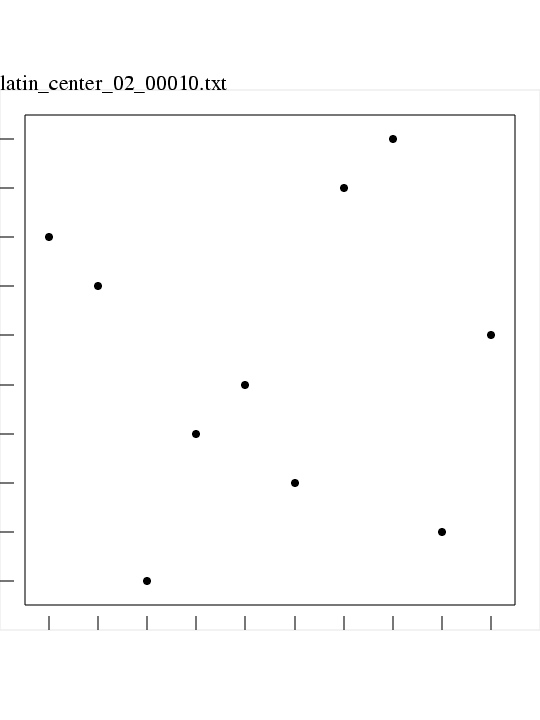# LATIN_CENTER Latin Center Squares in M dimensions

LATIN_CENTER is a C++ library which makes Latin Center Squares.

A Latin square is a selection of one point from each row and column of a square matrix or table. In M dimensions, the corresponding item is a set of N points, where, in each dimension, there is exactly one point whose coordinates are in a given "column" or range of values. To emphasize the use of higher dimensions, these objects are sometimes called Latin hypersquares.

Once a Latin Square has been created, a Latin Center Square is determined by choosing the center of each subsquare.

Here is an image of a Latin centered grid with spatial dimension M = 2 and number of points N = 10.### Languages:

LATIN_CENTER is available in a C++ version and a FORTRAN90 version and a MATLAB version.

### Related Data and Programs:

BOX_BEHNKEN, a C++ library which computes a Box-Behnken design, that is, a set of arguments to sample the behavior of a function of multiple parameters;

CVT, a C++ library which computes points in a Centroidal Voronoi Tessellation.

FAURE, a C++ library which computes Faure sequences.

GRID, a C++ library which computes points on a grid.

HALTON, a C++ library which computes elements of a Halton Quasi Monte Carlo (QMC) sequence, using a simple interface.

HAMMERSLEY, a C++ library which computes elements of a Hammersley Quasi Monte Carlo (QMC) sequence, using a simple interface.

HEX_GRID, a C++ library which computes sets of points in a 2D hexagonal grid.

IHS, a C++ library which computes improved Latin Hypercube datasets.

LATIN_CENTER_DATASET, a C++ program which creates a Latin Center Hypercube dataset;

LATIN_COVER, a C++ library which produces N Latin squares which cover an NxN square, or NxN Latin cubes which cover an NxNxN cube.

LATIN_EDGE, a C++ library which computes Latin square data choosing the edge value.

LATIN_RANDOM, a C++ library which computes Latin square data choosing a random value in the square.

NIEDERREITER2, a C++ library which computes Niederreiter sequences with base 2.

NORMAL, a C++ library which computes elements of a sequence of pseudorandom normally distributed values.

SOBOL, a C++ library which computes Sobol sequences.

UNIFORM, a C++ library which computes uniform random values.

VAN_DER_CORPUT, a C++ library which computes van der Corput sequences.

### Reference:

1. Paul Bratley, Bennett Fox, Linus Schrage,
A Guide to Simulation,
Springer Verlag, pages 201-202, 1983.
2. C J Colbourn and J H Dinitz,
CRC Handbook of Combinatorial Design,
CRC, 1996.
3. Bennett Fox,
Algorithm 647:
Implementation and Relative Efficiency of Quasirandom Sequence Generators,
ACM Transactions on Mathematical Software,
Volume 12, Number 4, pages 362-376, 1986.
4. M D McKay, W J Conover, R J Beckman,
A Comparison of Three Methods for Selecting Values of Input Variables in the Analysis of Output From a Computer Code,
Technometrics,
Volume 21, pages 239-245, 1979.
5. Albert Nijenhuis and Herbert Wilf,
Combinatorial Algorithms,
ISBN 0-12-519260-6.
6. Herbert Ryser,
Combinatorial Mathematics,
Mathematical Association of America, 1963.

### List of Routines:

• GET_SEED returns a random seed for the random number generator.
• I4_MAX returns the maximum of two integers.
• I4_MIN returns the smaller of two integers.
• I4_SWAP switches two integer values.
• I4_UNIFORM returns a scaled pseudorandom I4.
• LATIN_CENTER returns center points in a Latin square.
• PERM_UNIFORM selects a random permutation of N objects.
• R4_ABS returns the absolute value of an R4.
• R4_NINT returns the nearest integer to an R4.
• TIMESTAMP prints the current YMDHMS date as a time stamp.

You can go up one level to the C++ source codes.

Last revised on 01 December 2006.